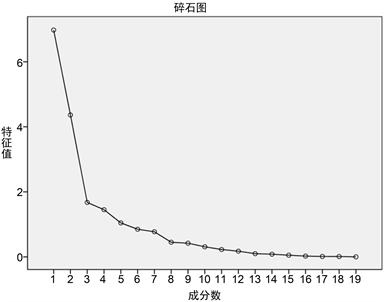﻿ 多发展指标下的各省人口因子与聚类分析

# 多发展指标下的各省人口因子与聚类分析Factor Analysis and Cluster Analysis of Province Population under Multiple Development Indexes

Abstract: With the increasing concern of environmental issues, people begin to think about the “population size and structure change in each region”. This paper uses factor analysis and cluster analysis to classify the population of each province and municipality directly under the Central Government. In this paper, the factor analysis method is used to build a comprehensive scoring model, including data standardization, factor extraction, naming and building a comprehensive model. This paper will select 31 provinces (autonomous regions and municipalities) under the Central Government data for factor analysis, through factor extraction combined with the cumulative contribution rate to determine five factors. Then the factor score formula (the relationship between the extracted factor F and each index X) and the comprehensive model score formula (the relationship between the comprehensive score Y and each index X) are obtained by calculation. Then the data are substituted into the constructed comprehensive scoring model to get the comprehensive scoring of each province and municipality directly under the Central Government and rank the comprehensive scoring. Then, the K-means clustering method is used to cluster the comprehensive scores of each city in one dimension, and the final result is that 31 provinces (autonomous regions and municipalities) under the Central Government are divided into six categories.

1. 研究意义

2. 人口发展水平因子分析

2.1. 数据标准化处理

$Z=\frac{X-\stackrel{¯}{X}}{\delta }$

2.2. 提取因子Table 1. Explained total varianceFigure 1. Scree plot

2.3. 因子命名

5个因子成分矩阵见表2

3. 各省份人口发展水平分析

3.1. 人口发展水平指标Table 3. Factor score coefficient matrix

3.2. 各省份各因子得分

Table 4. Factor score matrix

Table 5. Ranking of various factors

${\lambda }_{1}=6.978{\theta }_{1}=36.724%$

${\lambda }_{2}=4.365{\theta }_{2}=22.973%$

${\lambda }_{3}=1.675{\theta }_{3}=8.814%$

${\lambda }_{4}=1.451{\theta }_{4}=7.639%$

${\lambda }_{5}=1.044{\theta }_{5}=5.497%$

$\begin{array}{c}{F}_{1}=0.015{X}_{1}+0.139{X}_{2}+0.131{X}_{3}+0.133{X}_{4}+0.111{X}_{5}\\ \text{\hspace{0.17em}}\text{\hspace{0.17em}}+0.006{X}_{6}+0.001{X}_{7}+0.034{X}_{8}+0.034{X}_{9}+0.002{X}_{10}\\ \text{\hspace{0.17em}}\text{\hspace{0.17em}}+0.016{X}_{11}+0.006{X}_{12}+0.002{X}_{13}+0.135{X}_{14}+0.137{X}_{15}\\ \text{\hspace{0.17em}}\text{\hspace{0.17em}}+0.117{X}_{16}+0.016{X}_{17}+0.101{X}_{18}+0.115{X}_{19}\end{array}$

$\begin{array}{c}{F}_{2}=0.092{X}_{1}+0.019{X}_{2}+0.010{X}_{3}-0.060{X}_{4}-0.100{X}_{5}\\ \text{\hspace{0.17em}}\text{\hspace{0.17em}}-0.002{X}_{6}+0.053{X}_{7}-0.145{X}_{8}+0.084{X}_{9}+0.213{X}_{10}\\ \text{\hspace{0.17em}}\text{\hspace{0.17em}}+0.202{X}_{11}+0.193{X}_{12}-0.192{X}_{13}+0.018{X}_{14}+0.003{X}_{15}\\ \text{\hspace{0.17em}}\text{\hspace{0.17em}}-0.009{X}_{16}+0.087{X}_{17}+0.085{X}_{18}-0.002{X}_{19}\end{array}$

$\begin{array}{c}{F}_{3}=-0.204{X}_{1}-0.035{X}_{2}-0.088{X}_{3}+0.067{X}_{4}+0.129{X}_{5}\\ \text{\hspace{0.17em}}\text{\hspace{0.17em}}+0.391{X}_{6}+0.479{X}_{7}-0.034{X}_{8}-0.049{X}_{9}+-0.033{X}_{10}\\ \text{\hspace{0.17em}}\text{\hspace{0.17em}}+0.063{X}_{11}-0.101{X}_{12}-0.047{X}_{13}-0.071{X}_{14}-0.073{X}_{15}\\ \text{\hspace{0.17em}}\text{\hspace{0.17em}}+0.093{X}_{16}+0.300{X}_{17}-0.085{X}_{18}+0.071{X}_{19}\end{array}$

$\begin{array}{c}{F}_{4}=0.359{X}_{1}-0.058{X}_{2}-0.076{X}_{3}+0.061{X}_{4}+0.144{X}_{5}\\ \text{\hspace{0.17em}}\text{\hspace{0.17em}}+0.018{X}_{6}+0.178{X}_{7}+0.243{X}_{8}-0.267{X}_{9}+0.158{X}_{10}\\ \text{\hspace{0.17em}}\text{\hspace{0.17em}}+0.220{X}_{11}+0.174{X}_{12}+0.115{X}_{13}-0.095{X}_{14}-0.087{X}_{15}\\ \text{\hspace{0.17em}}\text{\hspace{0.17em}}+0.195{X}_{16}-0.341{X}_{17}-0.240{X}_{18}+0.135{X}_{19}\end{array}$

$\begin{array}{c}{F}_{5}=0.061{X}_{1}-0.121{X}_{2}+0.127{X}_{3}-0.026{X}_{4}+0.149{X}_{5}\\ \text{\hspace{0.17em}}\text{\hspace{0.17em}}-0.544{X}_{6}+0.213{X}_{7}-0.016{X}_{8}+0.550{X}_{9}-0.061{X}_{10}\\ \text{\hspace{0.17em}}\text{\hspace{0.17em}}-0.008{X}_{11}-0.066{X}_{12}+0.046{X}_{13}-0.163{X}_{14}-0.136{X}_{15}\\ \text{\hspace{0.17em}}\text{\hspace{0.17em}}+0.191{X}_{16}+0.185{X}_{17}-0.320{X}_{18}+0.168{X}_{19}\end{array}$

$Y=0.36724{F}_{1}+0.22973{F}_{2}+0.08814{F}_{3}+0.07639{F}_{4}+0.05497{F}_{5}$

4. 聚类分析

K-means算法是很典型的基于距离的聚类算法，采用距离作为相似性的评价指标，即认为两个对象的距离越近，其相似度就越大。该算法认为簇是由距离靠近的对象组成的，因此把得到紧凑且独立的簇作为最终目标。

${\mu }_{i1}=\frac{{y}_{i\mathrm{min}}-{y}_{i-1\mathrm{max}}}{{y}_{i\mathrm{max}}-{y}_{i\mathrm{min}}}$${\mu }_{i2}=\frac{{y}_{i\mathrm{min}}-{y}_{i-1\mathrm{max}}}{{y}_{i-1\mathrm{max}}-{y}_{i-1\mathrm{min}}}$$i=2,3,\cdots$

Table 7. Province clustering results

5. 结论

 路锦非, 王桂新. 我国未来城镇人口规模及人口结构变动预测[J]. 西北人口, 2010, 31(4): 1-6+11.

 国家数据[Z].
http://data.stats.gov.cn.

Top